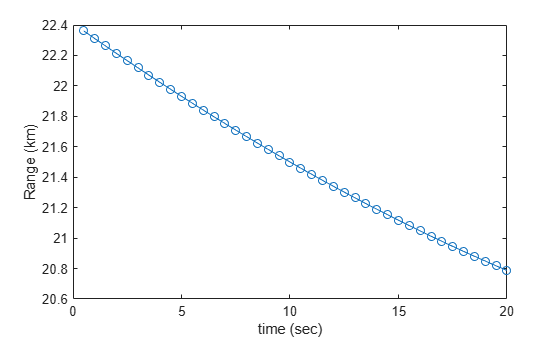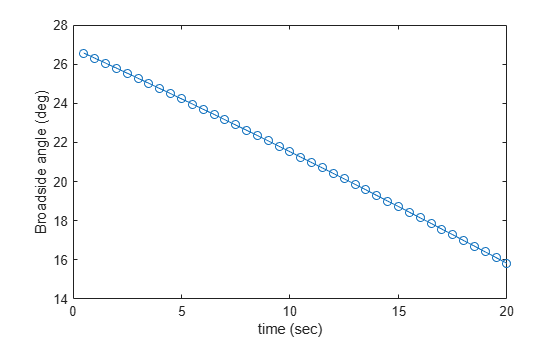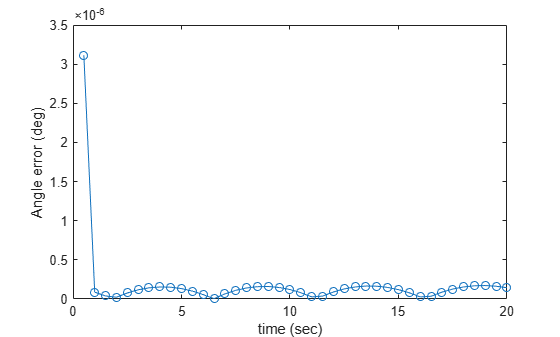# Target Tracking Using Sum-Difference Monopulse Radar

This example shows how to use the `phased.SumDifferenceMonopulseTracker` System object™ to track a moving target. The `phased.SumDifferenceMonopulseTracker` tracker solves for the direction of a target from signals arriving on a uniform linear array (ULA). The sum-difference monopulse algorithm requires a prior estimate of the target direction which is assumed to be close to the actual direction. In a tracker, the current estimate serves as the prior information for the next estimate. The target is a narrowband 500 MHz emitter moving at a constant velocity of 800 kph. For a ULA array, the steering vector depends only upon the broadside angle. The broadside angle is the angle between the source direction and a plane normal to the linear array. Any arriving signal is specified by its broadside angle.

### Create the target platform and define its motion

Assume the target is located at `[0,10000,20000]` with respect to the radar in the radar's local coordinate system. Assume that the target is moving along the y-axis toward the radar at 800 kph.

```x0 = [0,10000,20000].'; v0 = -800; v0 = v0*1000/3600; targetplatform = phased.Platform(x0,[0,v0,0].');```

### Set up the ULA array

The monopulse tracker uses a ULA array which consists of 8 isotropic antenna elements. The element spacing is set to one-half the signal wavelength.

```fc = 500e6; c = physconst('LightSpeed'); lam = c/fc; antenna = phased.IsotropicAntennaElement('FrequencyRange',[100e6,800e6],... 'BackBaffled',true); array = phased.ULA('Element',antenna,'NumElements',8,... 'ElementSpacing',lam/2);```

Assume a narrowband signal. This kind of signal can be simulated using the `phased.SteeringVector` System object.

`steervec = phased.SteeringVector('SensorArray',array);`

### Tracking Loop

Initialize the tracking loop. Create the `phased.SumDifferenceMonopulseTracker` System object.

```tracker = phased.SumDifferenceMonopulseTracker('SensorArray',array,... 'PropagationSpeed',c,... 'OperatingFrequency',fc);```

At each time step, compute the broadside angle of the target with respect to the array. Set the step time to 0.5 seconds.

```T = 0.5; nsteps = 40; t = (1:nsteps)*T;```

Setup data vectors for storing and displaying results

```rng = zeros(1,nsteps); broadang_actual = zeros(1,nsteps); broadang_est = zeros(1,nsteps); angerr = zeros(1,nsteps);```

Step through the tracking loop. First provide an estimate of the initial broadside angle. In this simulation, the actual broadside angle is known but add an error of five degrees.

```[tgtrng,tgtang_actual] = rangeangle(x0,[0,0,0].'); broadang0 = az2broadside(tgtang_actual(1),tgtang_actual(2)); broadang_prev = broadang0 + 5.0; % add some sort of error```
1. Compute the actual broadside angle, `broadang_actual`.

2. Compute the signal, `signl`, from the actual broadside angle, using the `phased.SteeringVector` System object.

3. Using the `phased.SumDifferenceMonopulseTracker` tracker, estimate the broadside angle, `broadang_est`, from the signal. The broadside angle derived from a previous step serves as an initial estimate for the current step.

4. Compute the difference between the estimated broadside angle, `broadang_est`, and actual broadside angle, `broadang_actual`. This is a measure of how good the solution is.

```for n = 1:nsteps x = targetplatform(T); [rng(n),tgtang_actual] = rangeangle(x,[0,0,0].'); broadang_actual(n) = az2broadside(tgtang_actual(1),tgtang_actual(2)); signl = steervec(fc,broadang_actual(n)).'; broadang_est(n) = tracker(signl,broadang_prev); broadang_prev = broadang_est(n); angerr(n) = broadang_est(n) - broadang_actual(n); end```

### Results

Plot the range as a function of time showing the point of closest approach.

```plot(t,rng/1000,'-o') xlabel('time (sec)') ylabel('Range (km)')```Plot the estimated broadside angle as a function of time.

```plot(t,broadang_actual,'-o') xlabel('time (sec)') ylabel('Broadside angle (deg)')```A monopulse tracker cannot solve for the direction angle if the angular separation between samples is too large. The maximum allowable angular separation is approximately one-half the null-to-null beamwidth of the array. For an 8-element, half-wavelength-spaced ULA, the half-beamwidth is approximately 14.3 degrees at broadside. In this simulation, the largest angular difference between samples is

```maxangdiff = max(abs(diff(broadang_est))); disp(maxangdiff)```
``` 0.2942 ```

The angular separation between samples is less than the half-beamwidth.

Plot the angle error. This is the difference between the estimated angle and the actual angle. The plot shows a very small error, on the order of microdegrees.

```plot(t,angerr,'-o') xlabel('time (sec)') ylabel('Angle error (deg)')```